# Construction of The Game

Author:
hejaclk

## Construction of The Game

1)Construct the check box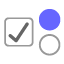is described "How to Play Game" 2)Construct a random button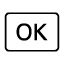to produce two random decimal numbers 3)Random button creates two variable called as "b" and "d" 4) Write ın random button script on click; b=RandomBetween(0,60) and d=RandomBetween(0,60) 5)Write two input called as c=b/10 and e=d/10 6)f is described f=|c-e| 7) Create two slider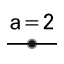"g" and "h" between 0 and 10 8)Write ın random button script on click If(f<0.5,SetValue(g,0),f<1.5,SetValue(g,1),f<2.5,SetValue(g,2),f<3.5,SetValue(g,3),f<4.5,SetValue(g,4),f<5.5,SetValue(g,5),f<6.5,SetValue(g,6),SetValue(g,7)) 9)Create the input box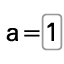and write in script on click If(g==h, SetValue(metin3,"push the start button"), SetValue(metin3,"opss, you can't play, now"))
10) Construct the start buttonin order to show "the time" by constructing the slider11)Write "StartAnimation[i,true] k=false" in script on click of start button 12)"i" is constructed as slider between 0 and 10 the velocity is 0.2 13)In order to construct the TANGRAM choose 4 points on coordinate system and draw a square 14)Draw geometric shapesas square, triangles, rhombus, parallelogram and right angles in the square.
15)Select the rigid poligon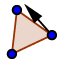and draw a certain number of geometric shapes as square, right angles, triangles, rhombus. 16)Construct a text boxfor the number of each of geometric shapes 17)Construct the "up & down" buttonunder each of the geometric shapes 18)Construct the slideras geometric shapes to be used. 19)Write the SetValue(written number in slider, written number in slider -1) in the script on click of "up & down" button 20)Repeat 16th, 17th, and 18th steps for each geometric shapes.